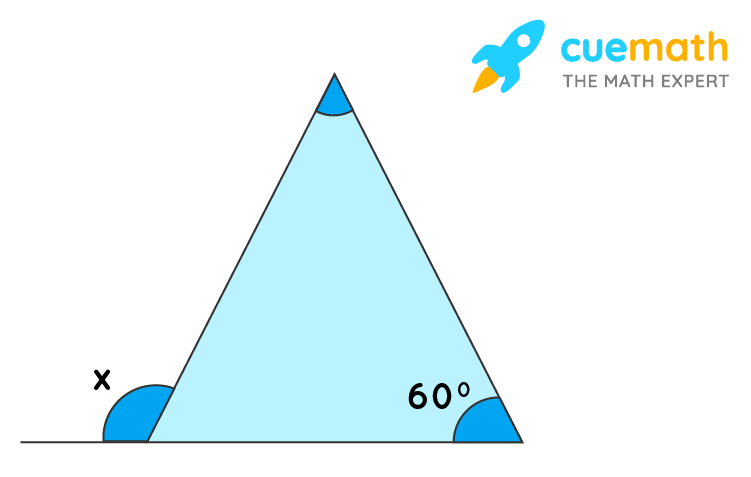# How to Find the Value of X in a Triangle

## Question: How to find the value of x in a triangle?A three-sided polygon is known as a triangle.

## Answer: The value of x in a triangle is 120°

Let us understand the concept through an example.

## Explanation

The given triangle is an equilateral triangle.

Hence, all angles are equal to 60°.

Now, for a triangle we know that

Interior angle + Exterior angle = 180°

60° + x = 180°

x = 180° –  60° = 120°.